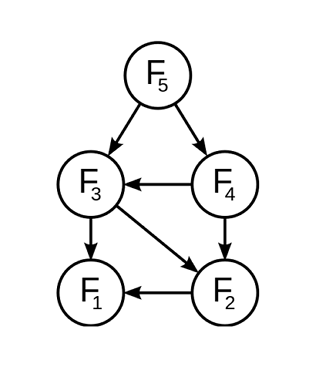# Fibonacci Power!How many values of $k$ are there such that $F_k$ is of the form $2^n$?

Note that both $k$ and $n$ are non-negative integers.

Also $F_0=0,F_1=1$ and $F_m=F_{m-1}+F_{m-2}$

×